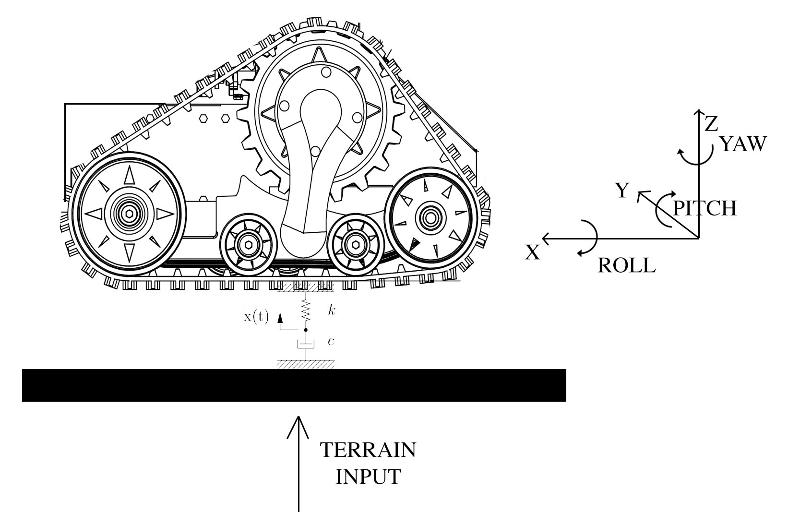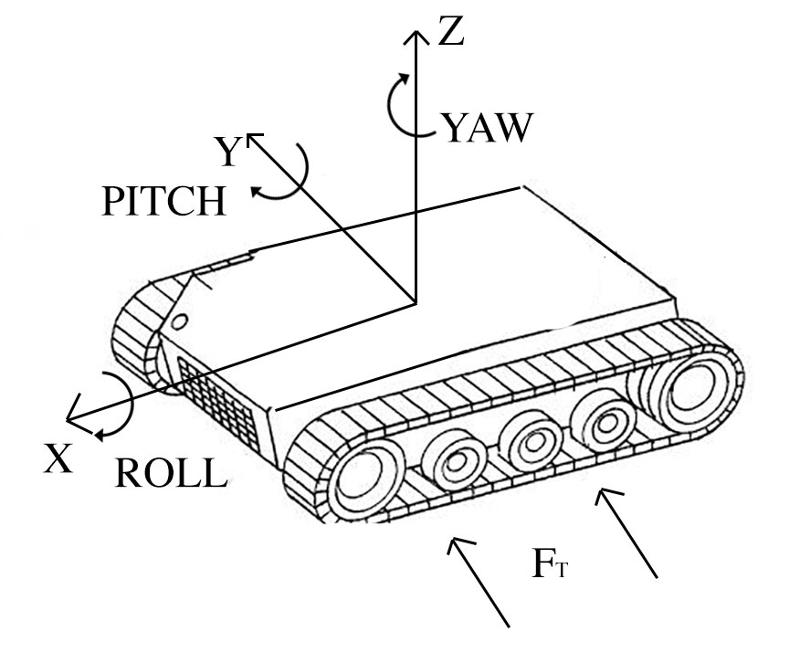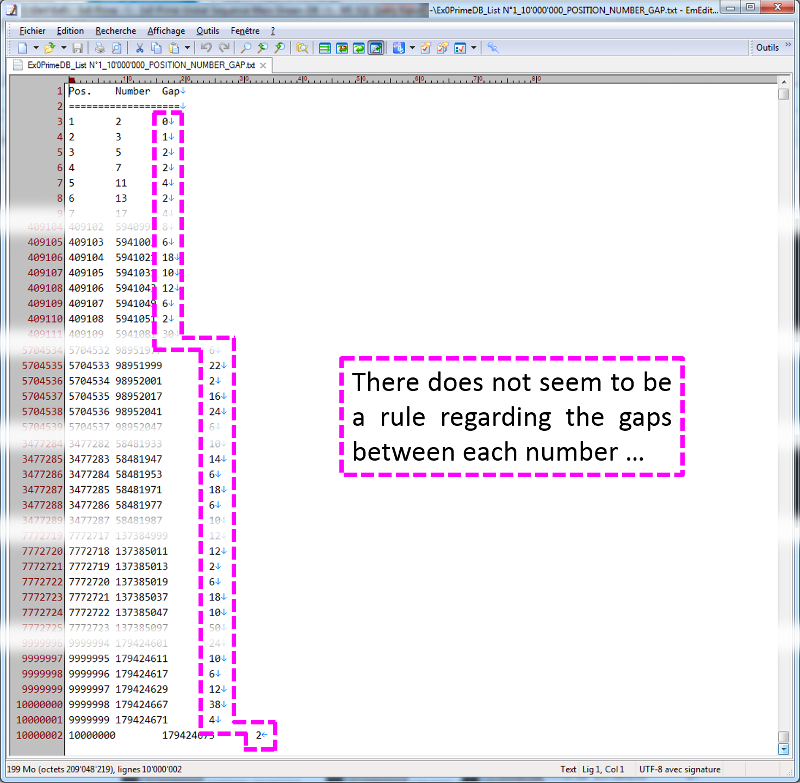Math / Science

9K

Solutions

6K

Contributors

The Math / Science topic primarily includes discussions of mathematics, physics, statistics and economic analysis, but also biology, chemistry and other sciences.

Share tech news, updates, or what's on your mind.
I would like to distribute the difference between the weekly labor target G2 and the weekly hours of operation I2 in even 0.25 chunks. The difference between G2 and I2 is in J2. If you multiply J2 by four you can see that I have 82 0.25 hour chunks of time available to distribute. How can I distribute those 82 chunks based on the needs represented by the ticket counts (workload) represented in column F. It's okay for some rows to be blank as long as I don't exceed the 82 chunks of time available and distribute the 0.25 hours to the intervals where the needs are highest.

I have tried distributing the labor based on a ratio defined by F/G but that often results in uneven labor or labor that is blowing the budget available.
Example_LaborDistribution.xlsx
0
what are good science experiments for my grade
0
I'd like to write a Lambda function that fetches a (1) CloudWatch Metric (that is already being monitored at every 5 minutes), (2) divides that value by 300 seconds and (3) pushes to CloudWatch as a Custom Metric.

The CloudWatch metrics already being monitored are "Volume Read Ops" and "Volume Write Ops" on a could different active EBS volumes.

I have no every on using Java or Python for this.

Thanks for your help or pointing me in the right direction.
0
I have a set of seven parameters which are numerical values (i.e. current readings, pitch, roll and yaw angles, acceleration, etc..) and I use them to classify a vehicle behavior.
For example, if:

current=5A
pitch=12 degrees
yaw=41 degrees
Y_acc = 10 m/s^2
...

then the vehicle behaviour is classified as A otherwise if

current=7A
pitch=15 degrees
yaw=42 degrees
Y_acc = 11 m/s^2
...

then the vehicle is classified as B, and so on..

What is the best way to implement this kind of classification in Matlab?
Should I use a simple neural network? Or a classification method like SVM?
Is there any tutorial or example?

I have a set of data where each set of parameters is related to a specific behavior, so I can use this to train my model.

Thank you!
0
why is aluminum foil good at keeping things cold
0
I need help finding the general mathematical form (similar to "n choose k") of a counting/probability problem. It arose after a coworker implemented a simple JS fix to prevent something from displaying multiple times when sampled randomly and I wondered what the odds of it happening were. As formally as I could state it is as follows:

Problem Statement:
Given n distinct options with equal probability of occurring, what is the probability of at least one grouping of type ni of size k or greater appearing after s independent samplings?

I've looked into multinomial coefficients but couldn't figure out how to generalize it so it didn't matter which pair happened or that larger pairs should count towards it. A Poisson Distribution also looked promising but the simplest form seems to only be concerned with the probability of a single thing happening over a given time frame. Instead I hacked together some JavaScript to at least get a sense of its behavior.

Example
Roll a die 10 times. What is the probability that any of the numbers occurs at least 3 times?

Monte Carlo Solution
This will happen approximately 93% of the time given by the following code:

function
0
Nick and Sarah are partners. Their invested capital is \$30000 and \$45000. Respectively

a)what is their capital ratio
b)if the share of each in a net profit of \$18000
0
he cut some apples into eighths. she and her friends ate all but 17 pieces. how many whole apples and parts of apples did she have left over? tell how you know?
0
Dear experts,

I am planning to pursue MS in Financial Engineering.

I come with a commerce background and do not have statistics background.

I am looking for anyone/online course which can teach me college level statistics. Please find attached the front page of the book which i intend to cover. I am looking for a resource which can teach me the subject.

I live in Visakhapatnam, India.

Kindly guide.

Thank you
statistics.jpg
0
I need to prove that certain patterns were engineered and not random events and have been told that Neural Networks can be used to help make this determination.

What kinds of tools and algorithms should I be looking at?

Any sample projects that have done something similar?
0
I had this question after viewing Google earth optimal conditions..

Okay google earth is primary video card related.

I have a follow up question.
If most expensive video card is purchased will it be better than second most expensive video card?

Is this called
big o
notation
where I need to figure out what video card will result in performance increase and how much increase that will be?
0
I am working on a college science presentation, with historical contexts, and I absolutely must have YBCO wire, I know there are other HTS wire out there, but due to the context, historical significance of YBCO, and research involved, I must have YBCO wire
0
Hi to all,

I would like to be able to write equations to model my tracked vehicle which has a mass m=200Kg.
My skid-steering vehicle uses one track per side with a rubber belt made in 100% natural rubber, please refer to the next photo to get a more detailed idea.Since I mounted an IMU in its geometric center, I can read  both the angular velocites and the accelerations along the three axis plus the heading, the pitch and yaw angles in degrees.

I would like to be able to write a model for the vehicle in order to simulate and study its outputs depending on specific inputs.

Each track has a length L=1000 mm, a width W=330 mm and a lug height, H = 32 mm.
The vehicle is Lv= 1000 mm long and Wv=900 mm width.
There are no suspensions, but I consider the rubber track as the combination of a spring, coefficient K, and a damper, coefficient b. The rubber compression is about Dx =1,5 mm

By supposing a sinusoidal input below the track generated by the terrain profile, u(t) = Asin(wt), I was able to write the equation for the Z axis:

X(s)/Y(S)=(bs+k)/((m/2)*s^2 + bs+k) for the right track
X(s)/Y(S)=(bs+k)/((m/2)*s^2 + bs+k) for the left track

Now, I would like to consider the lateral force Ft which acts on the robot during a turning motion.Let's consider the vehicle during a steering maneuver on the left: while the YAW angle …
0
Hello and Good Morning Everyone,

I am wondering how an Annual Percentage Rate (APR) of 26.70% be converted to a monthly rate.  Speaking hypothetically, I will put these numbers out here in order to capture or clarify my question.  An account has a balance of \$500 with an APR of 26.70%. Given this information, how much interest would be added to this balance within one month?

Thank you

George
0
I am trying to calculate the percentage of two column totals in Crystal Reports.  The formula below is the formula I am trying to use:

If {Command.cost}= 0 then 0 else sum{Command.cost}/sum{@labor gross}*100

What am I doing wrong?

0
Exactly that
0
Ok, so I am a beginner to trigonometry, and how do you convert a decimal to a degree? I dunno if i worded it dorrect, but a website said tan 0.5 is 26.6. I still duno if thsts correct, but if it is, someone explain in good detail on how they got 26.6 degrees? I know how to invert, tan,sin,and cos, coordinates,cortesian coordinates, perpendicular lines, right angles, reciprocals (correct me if im wrong, fraction interchanging?) So can someone simply explain how they got 26.6? Thanks.
0
hi to all,

I have a vector in Matlab which contains samples acquired at 10Hz from an accelerometer mounted on a frame's vehicle.
This vector contains the acceleration values along the Z-axis.
The acquisition time is 103 seconds.

I would like to plot the frequency values in Hz since I'm trying to study the terrain frequency response.

I tried to use the fft function available in Matlab, but I do not know how to proceed.
Can you help me, please?

These are the values contained in my vector:

size(vibration)
ans =
1024           1
vibration =
-0.0005
-0.0006
-0.0007
-0.0008
-0.0010
-0.0011
-0.0012
-0.0013
-0.0014
-0.0015
-0.0016
-0.0017
-0.0018
-0.0019
-0.0020
-0.0021
-0.0022
-0.0023
-0.0024
-0.0026
-0.0027
-0.0025
-0.0003
-0.0038
-0.0089
-0.0035
-0.0018
-0.0022
0.0004
0.0034
0.0063
-0.0023
-0.0046
-0.0022
-0.0043
0.0041
0.0025
0.0024
0.0037
0.0050
0.0060
0.0012
0.0058
0.0019
0.0008
0.0009
-0.0060
0.0004
0.0032
-0.0005
-0.0008
-0.0024
0.0021
0.0009
-0.0019
0.0003
0.0012
0.0069
0.0125
0.0209
0.0262
0.0184
0.0100
0.0058
0.0136
0.0181
0.0089
0.0058
0.0077
0.0045
0.0024
0.0015
0.0044
0.0095
0.0096
0.0087
0.0019
0.0053
0.0066
0.0033
-0.0010
0.0010
0.0116

0
Is it related to the fact that we do not know any rule regarding their distribution to date?

For example we have generated lists of several billion Consecutive Prime Numbers and it turns out that there does not seem to be a rule regarding the gaps between each number or at least not on an acceptable human scale .. ... .....Here you can download 2 of our small generated lists, the first one containing the first 10'000'000 and the second one containing the first 100'000'000 consecutive Prime Numbers all listed in the correct order with their exact positions, as well as the gap between each number.

Are there any available very long and detailled lists of Large Prime Numbers that allow for larger-scale analysis of the gaps between numbers ?

Or is there a mathematical rule to quantify those number gap or to know their emerging sequence?

Thank you in advance for your answers .. ... .....

Regards
0
Hello everybody,

It seems generally admitted that there are no negative prime numbers.

What are the rules that can affirm this?

Thanks in advance and happy new year to all.

Best regards,
0
I am looking for a single mathematical formula that takes in elevation, temperature, humidity and barometric pressure, and gives the speed of sound as an answer. I have found many simplified formulae which use only one variable to give an approximate answer but can't find one that provides a more accurate answer. I realize this may be out of the scope of experts exchange, but thought it can't help to ask.
0
Hi to all,

I have a vector " imu" with a size of 11497 elements. These elements are samples captured at a very high rate (the total time is 71 seconds so I think the frequency is about 160 Hz). Since I acquired all the other values at 10Hz, the size of all my other vectors is 719.

I would like to remove the oversampled elements from the vector " imu" in order to have only 10 samples per second.

Is there any way to do this? Can you help me, please?
0
Hi to all,

i'm having a problem with one of my old matlab scripts.
This script worked very well on a previous version of Matlab, but now I'm running it on a different machine and on a different Matlab version (R2017b) and it gives me this error:

Index exceeds matrix dimensions.
Error in cusum (line 36)
y   = x1(:,c_y) - mean(x1(:,c_y));
Error in original (line 269)
[ckc,slc]=cusum(Ic(:,2)); %% PROBLEMA

Please, can you try to help me?
I attached to this post all the matlab files (i renamed them with *.txt extension) and also the txt file to use to populate the vectors.

The main matlab file is 'original.m':

clear all, close all
%
% figure(1)
% set(1,'units','normalized','position',[0.142187 0.484722 0.307813 0.447222],....
%         'name','Path','numbertitle','off');
%     set(gca,'FontSize',11);
% figure(2)
% set(2,'units','normalized','position',[0.457031 0.484722 0.264063 0.447917],....
%         'name','Equivalent track','numbertitle','off');
%     set(gca,'FontSize',11);
%
% figure(3)
% set(3,'units','normalized','position',[0.009 0.13 0.482031 0.806944],....
%         'name','Terrain estimation','numbertitle','off');
%     set(gca,'FontSize',11);
%
% figure(4)
% set(4,'units','normalized','position',[0.00351563 0.0465278 0.214453 0.893056],....
%         'name','Path','numbertitle','off');

% First campaign january 2015
0
Suppose I need quantity Q grams of honey, and I can buy packs of honey in either 340g or 454g pack sizes. These packs cost 1.79 and 2.19 respectively (cost per kilo is therefore 5.26 and 4.82 respectively)

I buy m packs of 340g and n packs of 454g

Question: express m & n in terms of Q in order to minimise cost for any given Q.

I believe this is a solution: https://www.experts-exchange.com/questions/29063883/Optimum-pack-size-purchases-follow-up-to-minimum-cost-solution.html

But this maybe overbaking it (the least common multiple of 340 and 454 is 77180)

Wandering if I'm missing a shortcut somewhere.

Thanks
0
Dear experts,

In the attached image I have the Principal solution for positive and negative sin theta. The image shows how the negative theta values are reflected as positive alpha values.

Can someone please guide me to an article in public domain for equivalent workings of Cot, cosecant and secant theta.

Thank you
Principal-Solution-for-pos-and-neg-S.pdf
0

Math / Science

9K

Solutions

6K

Contributors

The Math / Science topic primarily includes discussions of mathematics, physics, statistics and economic analysis, but also biology, chemistry and other sciences.

Top Experts In
Math / Science
Monthly